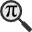## General

Display information for equation id:math.236899.57 on revision:236899

* Page found: Old quantum theory (eq math.236899.57)

(force rerendering)

Cannot find the equation data in the database. Fetching from revision text.

Occurrences on the following pages:

Hash: 7b7f9dbfea05c83784f8b85149852f08

TeX (original user input):

\alpha


TeX (checked):

\alpha


### LaTeXML (experimental; uses MathML) rendering

MathML (482 B / 255 B) :${\displaystyle\alpha}$
<math xmlns="http://www.w3.org/1998/Math/MathML" id="p1.1.m1.1" class="ltx_Math" alttext="{\displaystyle\alpha}" display="inline">
<semantics id="p1.1.m1.1a">
<mi id="p1.1.m1.1.1" xref="p1.1.m1.1.1.cmml">α</mi>
<annotation-xml encoding="MathML-Content" id="p1.1.m1.1b">
<ci id="p1.1.m1.1.1.cmml" xref="p1.1.m1.1.1">𝛼</ci>
</annotation-xml>
<annotation encoding="application/x-tex" id="p1.1.m1.1c">{\displaystyle\alpha}</annotation>
</semantics>
[/itex]


SVG (1.132 KB / 681 B) :

### MathML with SVG or PNG fallback (recommended for modern browsers and accessibility tools) rendering

SVG image empty. Force Re-Rendering

SVG (0 B / 8 B) :

PNG (0 B / 8 B) :

$\alpha$## Translations to Computer Algebra Systems

### Translation to Maple

In Maple: alpha

\alpha: Could be the second Feigenbaum constant.

But this system don't know how to translate it as a constant. It was translated as a general letter.


### Translation to Mathematica

In Mathematica: \[Alpha]

\alpha: Could be the second Feigenbaum constant.

But this system don't know how to translate it as a constant. It was translated as a general letter.


## Similar pages

Calculated based on the variables occurring on the entire Old quantum theory page

## Identifiers

• $\alpha$### MathML observations

0results

0results

no statistics present please run the maintenance script ExtractFeatures.php

0 results

0 results